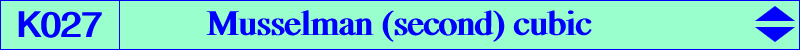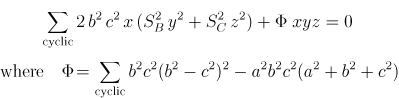X(4), X(10412), X(14380), X(15329), X(43665), X(43728), X(53153), X(53154), X(53175), X(53176), X(53177), X(53178), X(56742), X(56881), X(58070), X(58071), X(58072) infinite points of the Kjp cubic K024 imaginary foci of the MacBeath inconicThis cubic is cK60(#X4, X2052) with node H and nodal tangents parallel to the asymptotes of the circum-conic with perspector X(800), passing through X(110), X(1624). It is (partially) described in Musselman's paper (see bibliography) in the following manner. Denote by A2, B2, C2 the reflections of P in the sidelines BC, CA, AB and by A3, B3, C3 the reflections of H in the lines AP, BP, CP. The three circles AB2C2, BC2A2, CA2B2 have a common point N on the circumcircle. The three circles PAA3, PBB3, PCC3 have P in common and another point L also on the circumcircle. These points N, L coincide if and only if P lies on the Musselman (second) cubic K027 and they are antipodes if and only if P lies on the Musselman (third) cubic K028 (Musselman, Some loci connected with a triangle. Monthly, p.354-361, June-July 1940). K027 is more simply the locus of the intersection of the tangent at M and the Steiner line of M when M traverses the circumcircle of ABC. K027 is tritangent to the circumcircle at A, B, C. The triangle formed by the asymptotes is centered at X(381), the midpoint of X(2), X(4). Its three asymptotes are parallel to the sidelines of the Morley triangle. The isogonal transform of K027 is K1320.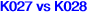Recall that K027 and K028 are both equilateral cubics which are not of the same type but nevertheless they share some similar properties. K028 is a psK meeting the sidelines of ABC at the vertices A', B', C' of a cevian triangle (that of X264) and K027 is a cK meeting these sidelines at three collinear points U, V, W on the trilinear polar of its root X(2052). The asymptotes of K027, K028 are parallel to those of K024, K003 respectively. Those of K028 concur at X(381) which turns out to be the center of the equilateral triangle formed by those of K027. K027 and K028 meet at nine (finite) points which are A, B, C, H (counting for four) and two other imaginary isogonal conjugates points P1, P2 lying on the perpendicular bisector of OH and on the circum-conic (C50). These two points are actually the imaginary foci of the McBeath inconic. They also lie on K005 and on any isogonal focal nK which is the locus of foci of inconics with center on a line passing through X(5). Their roots lie on the trilinear polar of X(264) and their foci lie on the circumcircle. See Table 80.Each cubic must meet the perpendicular bisector of OH (i.e. the trilinear polar of X94) again at a real point P27, P28 respectively. • P27 lies on the lines 4,1510 - 53, 2501 with SEARCH = -0.442699845350896. • P28 lies on the lines 2,1138 - 3,476 - 4,94 with SEARCH = 0.0941046961049146. P27, P28 are the barycentric quotients of X(1989) and X(110), X(74) respectively and also the barycentric products of X(94) and X(523), X(30) respectively. P27, P28 are now X(10412), X(14254) in ETC.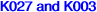We know that the isogonal conjugates of the infinite points of K003 are the vertices of an equilateral triangle, namely the CircumNormal triangle N1N2N3. More generally, for any pole Ω on the circum-conic (C51) with perspector X(51), the Ω-isoconjugates of these same infinite points are also the vertices of an equilateral triangle S1S2S3 whose center S lies on K027. The sidelines of this triangle are perpendicular to the sidelines of the CircumNormal triangle.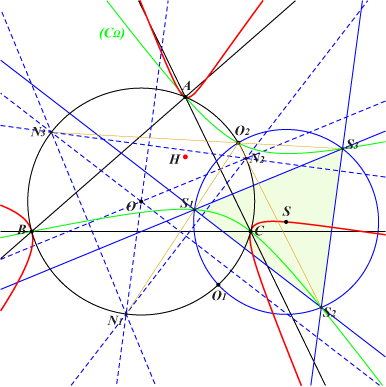This triangle S1S2S3 is obviously inscribed in the circum-conic (CΩ) which is the Ω-isoconjugate of the Ω.line at infinity. (CΩ) meets the circum-circle (O) again at O2, the trilinear pole of the line X(6), Ω. The circum-circle of S1S2S3 is the circle with center S that passes through O2. This cicle is orthogonal to (O) and meets (O) again at O1, the barycentric quotient Ω ÷ X(5). The isogonal transform of (CΩ) is a line (LΩ) tangent to the in-conic with center X(140) which is also inscribed in the CircumNormal triangle N1N2N3. Note that these triangles N1N2N3 and S1S2S3 are perspective at O2. *** See K028 and K024 for very similar properties.